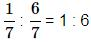Courses

# Test: Alligation Or Mixture- 2

## 10 Questions MCQ Test Quantitative Aptitude (Quant) | Test: Alligation Or Mixture- 2

Description
This mock test of Test: Alligation Or Mixture- 2 for Quant helps you for every Quant entrance exam. This contains 10 Multiple Choice Questions for Quant Test: Alligation Or Mixture- 2 (mcq) to study with solutions a complete question bank. The solved questions answers in this Test: Alligation Or Mixture- 2 quiz give you a good mix of easy questions and tough questions. Quant students definitely take this Test: Alligation Or Mixture- 2 exercise for a better result in the exam. You can find other Test: Alligation Or Mixture- 2 extra questions, long questions & short questions for Quant on EduRev as well by searching above.
QUESTION: 1

Solution:
QUESTION: 2

### A vessel is filled with liquid, 3 parts of which are water and 5 parts of syrup. How much of the mixture must be drawn off and replaced with water so that the mixture may be half water and half syrup?

Solution:

According to the question, Ratio of initial mixture will be same as ratio of mixture after taken out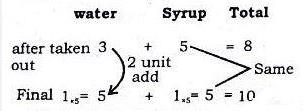Mixture is taken out

Initial mixture = 8+2=10

taken out = 2

=2/10=1/5

QUESTION: 3

### A container contains a mixture of two liquids P and Q in the ratio 7 : 5. When 9 litres of mixture are drawn off and the container is filled with Q, the ratio of P and Q becomes 7 : 9. How many litres of liquid P was contained in the container initially?

Solution: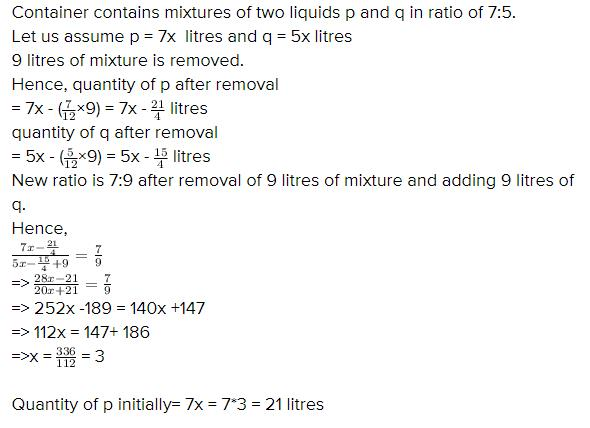QUESTION: 4

In what ratio must a grocer mix two varieties of pulses costing Rs.15 and Rs. 20 per kg respectively to obtain a mixture worth Rs.16.50 per Kg?

Solution:
QUESTION: 5
In what ratio must tea at Rs.62 per Kg be mixed with tea at Rs. 72 per Kg so that the mixture must be worth Rs. 64.50 per Kg?

Solution:
QUESTION: 6
A dishonest milkman sells his milk at cost price but he mixes it with water and thereby gains 25%. What is the percentage of water in the mixture?

Solution:
QUESTION: 7
In what ratio must tea worth Rs. 60 per kg be mixed with tea worth Rs. 65 a Kg such that by selling the mixture at Rs. 68.20 a Kg ,there can be a gain 10%?

Solution:
QUESTION: 8
A merchant has 1000 kg of sugar part of which he sells at 8% profit and the rest at 18% profit. He gains 14% on the whole. The Quantity sold at 18% profit is

Solution:
QUESTION: 9
In what ratio must water be mixed with milk costing Rs.12 per litre in order to get a mixture worth of Rs.8 per litre?

Solution:
QUESTION: 10

In what ratio must water be mixed with milk to gain 16 2/3 % on selling the mixture at cost price?

Solution:

Let C.P. of 1 litre milk be Rs. 1
S.P. of 1 litre of mixture = Rs. 1, Gain =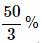∴ C.P. of 1 litre of mixture =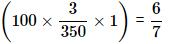BY the rule of alligation, we have: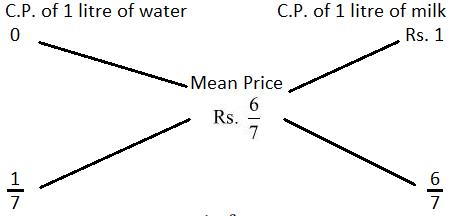∴ Ration of water and milk =